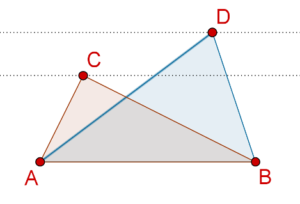# Same Base, Same Parallels

Go back to  'Triangles-Quadrilaterals'

Consider the following figure, which shows two parallelograms ABCD and ABEF on the same base AB: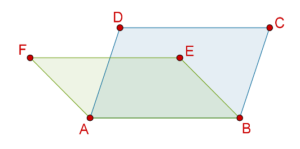Here’s another example. In the following figure, parallelogram ABCD and triangle ABE are on the same base AB: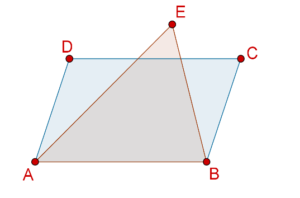Now, consider the following figure, in which two parallelograms ABCD and ABEF are on the same base as well as between the same parallels: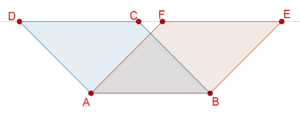Finally, consider the following figure, in which parallelogram ABCD and triangle ABE are on the same base as well as between the same parallels: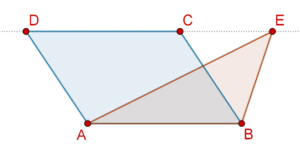We see that two figures can be said to be on the same base and between the same parallels if they have a common base (a common side) and the vertex (or vertices) opposite to the common base of each figure lie on some line parallel to the base.

In the following figure, the two triangles are on the same base but not between the same parallels: